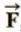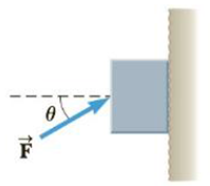Chapter 5, Problem 76AP

Chapter
Section
Textbook Problem

A 5.0-kg block is pushed 3.0 m up a vertical wall with constant speed by a constant force of magnitude F applied at an angle of θ = 30° with the horizontal, as shown in Figure P5.76. If the coefficient of kinetic friction between block and wall is 0.30, determine the work done by (a), (b) the force of gravity, and (c) the normal force between block and wall, (d) By how much does the gravitational potential energy increase during the block’s motion?Figure P5.76

(a)

To determine
The work done by the force F .

Explanation

Section1:

To determine: To determine the force F .

Answer: The force F is 2.0×102N .

Explanation:

Given Info:

The mass of the block is 5.0kg .

The distance that the block is pushed up is 3.0m .

The angle to which the force is applied is 30.0° .

The coefficient of kinetic friction between the block and the wall is 0.30 .

Since, the block id moving with constant speed,

Fx=0

Fy=0

The force of friction is,

fk=μkn

• μk is the coefficient of kinetic friction
• n is the normal force

Since, the normal force is,

n=Fcosθ

Therefore,

fk=μk(Fcosθ)

Consider the direction motion of the block to up is positive, then from the free body diagram,

Fsinθ=mg+μkFcosθ

On re-arranging,

Formula to calculate force is,

F=mgsinθμkcosθ

Substitute 5.0kg for m, 9.8m/s2 for g, 30.0° for θ and 0.30 for μk to find the force,

F=(5

(b)

To determine
The work done by force of gravity.

(c)

To determine
The work done by the normal force between the block and the wall.

(d)

To determine
The increase in gravitational potential energy during the block’s motion.

Still sussing out bartleby?

Check out a sample textbook solution.

See a sample solution

The Solution to Your Study Problems

Bartleby provides explanations to thousands of textbook problems written by our experts, many with advanced degrees!

Get Started

Find more solutions based on key concepts